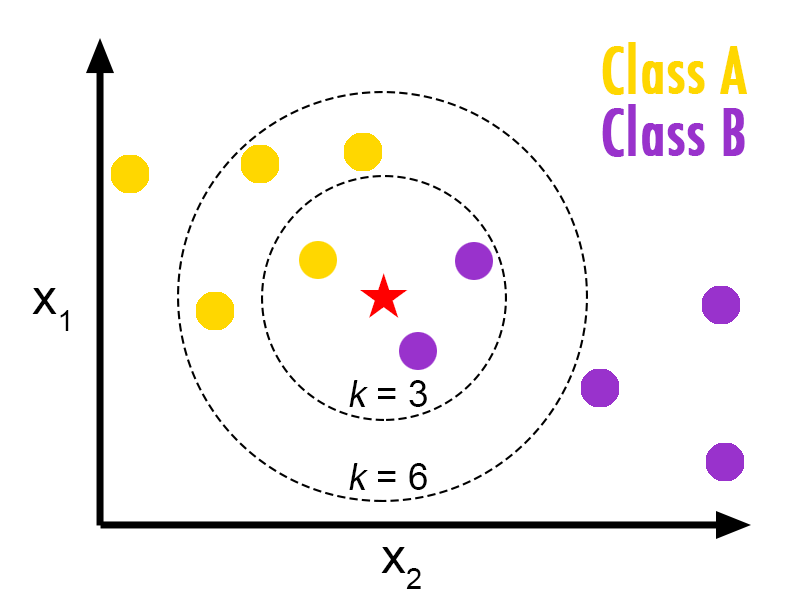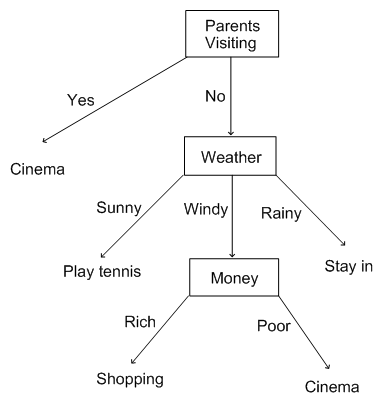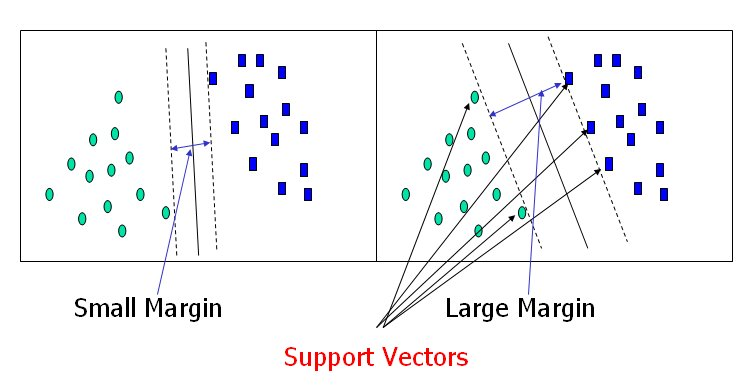Journey Details# Classification Algorithms

Classification is a technique to determine which fixed set of classes does an object belongs to. There are 2 steps involved in classification:

• Learning
• Induce classifiers from training data
• Predicting
• Classify objects using the classifier

## One R

A One R classifier generates a set of rules that all test on one particular attribute. It builds one rule for each attribute in the training data and then selects the rule with the smallest error. It treats all numerically valued features as continuous and uses a straightforward method to divide the range of values into several disjoint intervalsHow to choose the attribute to be used for the rule:

• For each attribute
• For each value of attribute:
• Count how often each class appears. Find most frequent class, and make the rule assign that class to this attribute-value
• Calculate the error rate of the rules
• Choose rules with the smallest error rate

• Most Simple/Primitive Algorithm
• Easy to implement and understand

• produces simple rules based on one feature only

## K-Nearest Neighbor

K nearest neighbor is a classification algorithm in which the nearest neighbor of an object is calculated on the basis of value of k, that specifies how many nearest neighbors are to be considered to define class of a sample data point.

Finds a group of k objects in the training set that are closest to the test object, and bases the assignment of a label on the predominance of a particular class in this neighborhood. There are three key elements of this approach: a set of labeled objects, e.g., a set of stored records, a distance or similarity metric to compute distance between objects, and the value of k, the number of nearest neighbors.To classify an unlabeled object, the distance of this object to the labeled objects is computed, its k-nearest neighbors are identified, and the class labels of these nearest neighbors are then used to determine the class label of the object.

• It is an easy to understand
• Training is very fast, because KNN classifiers are lazy learners

• One is the choice of k. If k is too small, then the result can be sensitive to noise points. On the other hand, if k is too large, then the neighborhood may include too many points from other classes.
• Memory limitation.
• Slow evaluation, because KNN classifiers are lazy learning algorithm

## Decision Tree

A decision tree is a flow-chart-like tree structure, where the internal node denotes a test on an attribute, the branch represents an outcome of the test, and the leaf nodes represent class labels or class distribution.The Decision Tree consists of 2 phases:

• Tree construction
• At start, all the training examples are at the root. A decision tree is constructed by recursively partition data based on selected attributes into sub-groups. Select the attribute with the highest information gain.
• Tree pruning
• Pruning is a technique that reduces the size of decision trees by removing sections of the tree that provide little power to classify instances. Pruning reduces the complexity of the final classifier, and hence improves predictive accuracy by the reduction of overfitting.

• Easy-to-understand.
• Map nicely to a set of production rules.
• Able to process both numerical and categorical data.
• Comparatively faster than Neural Networks.

• Calculations can get very complex particularly if many values are uncertain and/or if many outcomes are linked.
• Output attribute must be categorical.
• Limited to one output attribute.
• Decision tree algorithms are unstable.

## Artificial Neural Network

Artificial Neural Network is a network inspired by biological neural networks.A neural network is a set of connected input/output units in which each connection has a weight associated with it. During the learning phase, the network learns by adjusting the weights so as to able to predict the correct class label of the input.

The Artificial Neural Network consists of 2 phases:

• Forward phase: In this phase input is presented and output is calculated based on activation function and finally error is calculated at outer layer. (input → inner layers → output)
• Backward phase: In this phase error is send back to the inner layers to adjust the weights. Also known as Backpropagation Algorithm, iteratively process a set of training tuples & compare the network's prediction with the actual known target value. (output → inner layers → get different weight for inputs)

• Capable of producing an arbitrarily complex relationship between input and output, as we can adjust the weight of each variable.
• High tolerance to noisy data.
• Well-suited for continuous-valued inputs and outputs.
• Successful on a wide array of real-world data.

• Do not work well when there are many hundreds or thousands of input features and difficult to understand the model.
• Long training time
• Don’t know how many neurons needed, or how to connect them.

## Support Vector Machines

The aim of SVM is to find the best classification function to distinguish between members of the two classes in the training data. The metric for the concept of the “best” classification function can be realized geometrically.

For a linearly separable dataset, a linear classification function corresponds to a separating hyperplane f (x) that passes through the middle of the two classes, separating the two. Once this function is determined, new data instance xn can be classified by simply testing the sign of the function f (xn); xn belongs to the positive class if f (xn) > 0.The best function is found by maximizing the margin between the two classes. The reason why SVM insists on finding the maximum margin hyperplanes is that it offers the best generalization ability. It allows not only the best classification performance (e.g., accuracy) on the training data, but also leaves much room for the correct classification of the future data.

• Less overfitting, robust to noise.
• Especially popular in text classification problems

• SVM is a binary classifier. Can only distinguish into 2 classes.
• Computationally expensive, thus runs slow. (High complexity)

### Reference:

Pinky S., P., Patel, R., J. Patel, A., & Joshi, M. (2015). Review on Classification Algorithms in Data Mining. International Journal of Emerging Technology and Advanced Engineering, 5(1), 593–595.

Wu, X., Kumar, V., Quinlan, J. R., Ghosh, J., Yang, Q., Motoda, H., … Steinberg, D. (2007). Top 10 algorithms in data mining.. doi:10.1007/s10115-007-0114-2